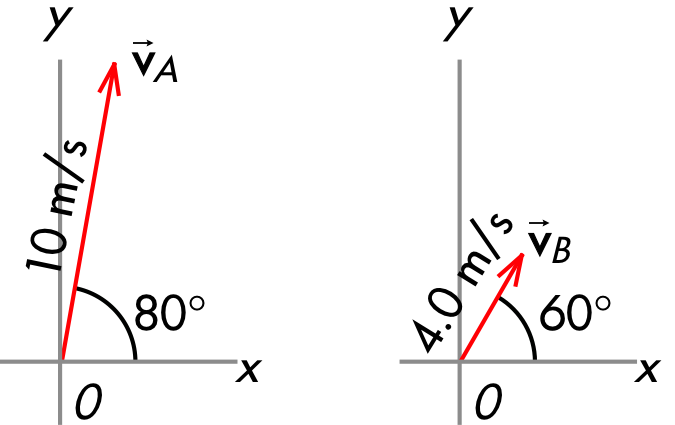## 20190924

### Physics quiz question: comparing horizontal velocity components

Physics 205A Quiz 3, fall semester 2019
Cuesta College, San Luis Obispo, CATwo velocity vectors shown at right have different speeds and directions. All angles are measured counterclockwise from the +x axis. Velocity vector __________ has the larger horizontal component magnitude.
(A) vA.
(B) vB.
(C) (There is a tie.)
(D) (Not enough information is given.)

Correct answer (highlight to unhide): (B)

Since these θ angles are measured counterclockwise from the +x axis, the horizontal components of these velocity vectors are given by:

vA,x = vA·cosθA,
vB,x = vB·cosθB.

Then the horizontal components are these velocity vectors can be calculated and compared:

vA,x = vA·cosθA = (10 m/s)·cos(80°) = 1.736481776669303... m/s,

or to two significant figures, the horizontal component of vA has a magnitude of 1.7 m/s, while:

vB,x = vB·cosθB = (4.0 m/s)·cos(60°) = 2.0 m/s.

Thus the horizontal component of vB is greater than the horizontal component of the horizontal component of vA.

Sections 70854, 70855
Exam code: quiz03Ch3V
(A) : 4 students
(B) : 49 students
(C) : 1 student
(D) : 0 students

Success level: 91%
Discrimination index (Aubrecht & Aubrecht, 1983): 0.19